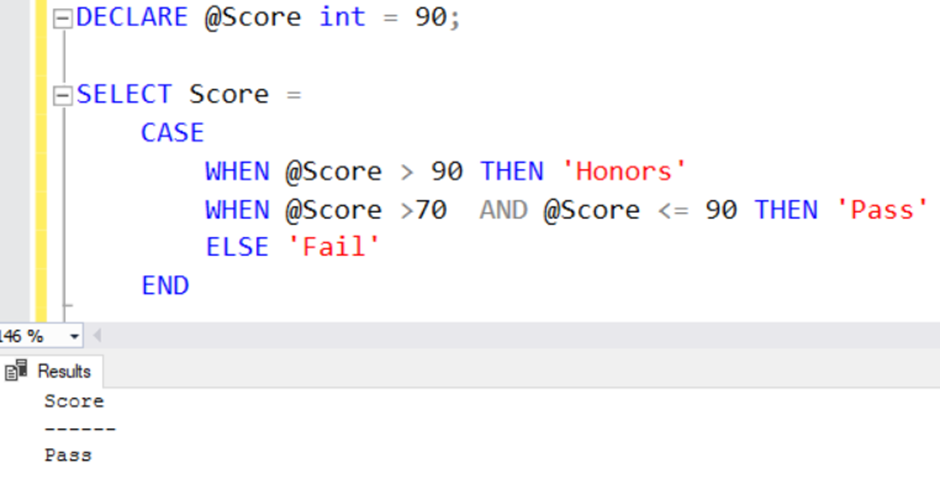top of page

# Learn about Case Statement in SQL Server

Case statements in SQL Server are used to provide instructions to be executed based on conditions. Case statements can be used in SELECT, INSERT, UPDATE, DELETE and MERGE statements.

A CASE expression in SQL Server (also called a "CASE statement") is used when you want to return one of many different results and manipulate them according to a given set of criteria. It's commonly used in SQL Server and works similarly across other databases.

The SQL Server CASE_expression evaluates a list of conditions and returns one of the multiple possible result expressions.

The Simple CASE Expression

Suppose we store countries short or popular names in SQL tables. However, we want to display their full name in the customer report. In this case, CASE STATEMENT IN SQL Server can help us.

Look at the following T-SQL. We evaluate the input value of the @CountryCode variable and define their corresponding values.

DECLARE @CountryCode varchar(10) = 'IND';

SELECT Country =

CASE @CountryCode

WHEN 'IND' THEN 'INDIA'

WHEN 'USA' THEN 'UNITED STATES OF AMERICA'

WHEN 'UK' THEN 'UNITED KINGDOM'

ELSE 'Wrong input'

END

For example, if the user input is IND, it evaluates the first expression and returns the value as INDIA.Let's look at another example where it returns the output as - Wrong input. Suppose we made a TYPO and misplaced the country short name as INDD. In this case, none of the case statement conditions were satisfied. Therefore, it reaches to ELSE condition and prints the message.

DECLARE @CountryCode varchar(10) = 'INDD';

SELECT Country =

CASE @CountryCode

WHEN 'IND' THEN 'INDIA'

WHEN 'USA' THEN 'UNITED STATES OF AMERICA'

WHEN 'UK' THEN 'UNITED KINGDOM'

ELSE 'Wrong input'

ENDSearched Case statement in SQL Server

In the searched case statement, SQL Server evaluates an expression; if the result satisfies a condition, it gives the result.

For example, let's say we want to print student results based on the score obtained. As shown below, the input score value is 90. It satisfies the 2nd condition (WHEN @Score >70 AND @Score <= 90 THEN 'Pass'). Therefore, you get the output as Pass.

DECLARE @Score int = 90;

SELECT Score =

CASE WHEN @Score > 90 THEN 'Honors'

WHEN @Score >70 AND @Score <= 90 THEN 'Pass'

ELSE 'Fail'

ENDSimilarly, if none of the conditions gets satisfied, it prints output as Fail.

DECLARE @Score int = 55;

SELECT Score =

CASE

WHEN @Score > 90 THEN 'Honors'

WHEN @Score >70 AND @Score <= 90 THEN 'Pass'

ELSE 'Fail'

ENDNote: SQL Server evaluates the case expression conditions sequentially. Once it finds a match, it stops processing the remaining conditions.

Both case expressions are satisfied in the following case statement in SQL Server. It prints the message – Honors and terminates the remaining expressions.

score 90 is available in both conditions. Therefore, once SQL Server starts processing the T-SQL, It checks for the first condition (when @score=90) and finds a match.

DECLARE @Score int = 90;

SELECT Score =

CASE

WHEN @Score =90 THEN 'Honors'

WHEN @Score >70 AND @Score <= 90 THEN 'Pass'

ELSE 'Fail'

END